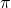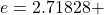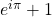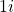Want to share your content on R-bloggers? click here if you have a blog, or here if you don't.In this post, we will first give some intuition for and then demonstrate what is often called the most beautiful formula in mathematics, Euler’s identity, in R – first numerically with base R and then also symbolically, so read on!

Euler’s identity (also known as Euler’s equation) is the equality:where

•is Euler’s number, the base of natural logarithms
•is the imaginary unit, which satisfies•is the ratio of the circumference of a circle to its diameter

It is often credited as the most beautiful formula in mathematics, nerds sport it on T-shirts and even get tattoos with it.

It combines three of the basic arithmetic operations: addition, multiplication, and exponentiation and links five fundamental mathematical constants:

• The number 0
• The number 1
• The number• The number• The numberthe imaginary unit of the complex numbers

We won’t go into the mathematical details here (when you google it you can find literally thousands of posts, articles, videos, and even whole books on it) but just give you some hand-waving intuition: as stated aboveis the ratio of the circumference of a circle to its diameter, which means that when you have a radius of 1 (= unit circle) you will needto go full circle. This is illustrated in the following animation:

Many of you know the exponential function (thanks to Covid anyway) which is nothing else but taking Euler’s numberto some power. Something magical happens when you take imaginary/complex instead of the “normal” real numbers: the exponential function starts to rotate:

As we have seen above a rotation byboils down to a rotation bydegrees. So when you start at 0and put that into the exponential function you get 1 (because) and when you then do a one-eighty (=) you will end up at -1. To get to the right-hand side of the identity you just have to add 1 to that -1 which equals 0. So Euler’s identity basically means:

When you turn around, you will look in the opposite direction!

Seen this way, it is easy, isn’t it!

Now for the R part. The following is the original task from Rosetta Code (for more solved Rosetta code tasks see the respective Category: Rosetta Code on this blog):

Show in your language that Euler’s identity is true. As much as possible and practical, mimic the Euler’s identity equation.

Most languages are limited to IEEE 754 floating point calculations so will have some error in the calculation.

If that is the case, or there is some other limitation, show thatis approximately equal to zero and show the amount of error in the calculation.

If your language is capable of symbolic calculations, show thatis exactly equal to zero for bonus kudos points.

First, as always, you should give it a try yourself……and now for the solution!

For coding the left-hand side of the identity we have to know the following:

•is not a built-in constant. Instead, the exponential function exp() is used (if you want to get Euler’s number just use exp(1))
• R can handle complex numbers! You can use the complex() function for that, or the Re() and Im() functions for the real and the imaginary parts. In this case it is even easier because we will only needand this is exactly the way we code it in R: 1i!
•is an built-in constant: pi

Putting it all together:

exp(1i * pi) + 1
##  0+1.224606e-16i


Besides the small rounding error, this is the whole solution!

Now for the symbolic solution to also get the bonus kudos points.

We will use the fantastic Ryacas package (on CRAN) to finish the job (for an introduction to this package see: Doing Maths Symbolically: R as a Computer Algebra System (CAS)).

library(Ryacas)
##
## Attaching package: 'Ryacas'
## The following object is masked from 'package:stats':
##
##     integrate
## The following objects are masked from 'package:base':
##
##     %*%, diag, diag<-, lower.tri, upper.tri

as_r(yac_str("Exp(I * Pi) + 1"))
##  0


And this finishes the task. It is also the solution I contributed to Rosetta code.

Isn’t maths beautiful! And isn’t R beautiful!